Ex 15.1

Chapter 15 Class 10 Probability
Serial order wise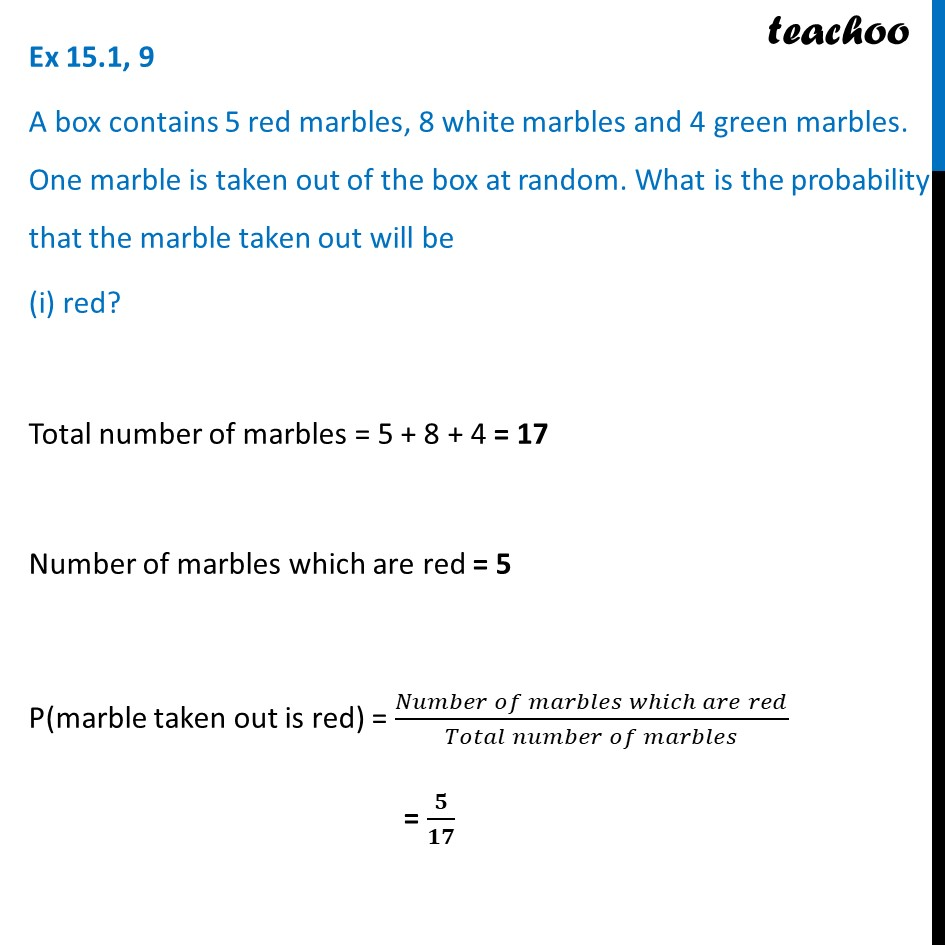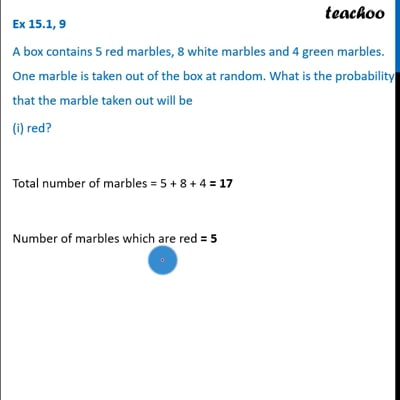This video is only available for Teachoo black users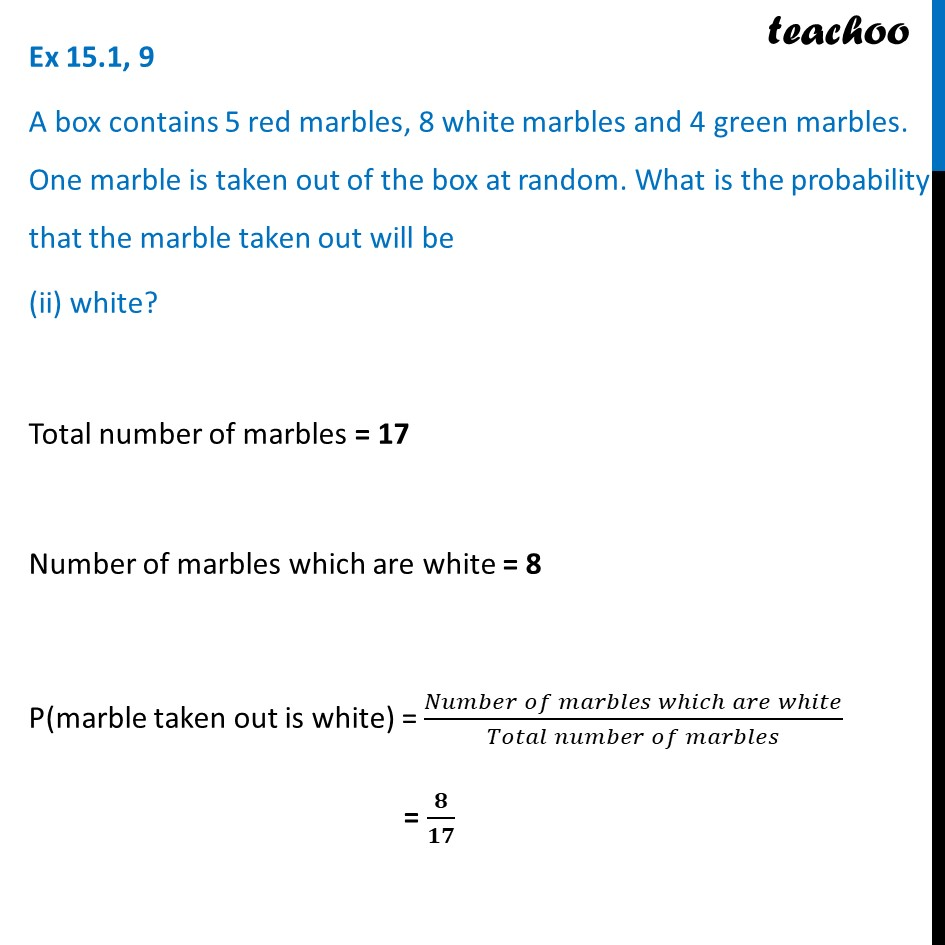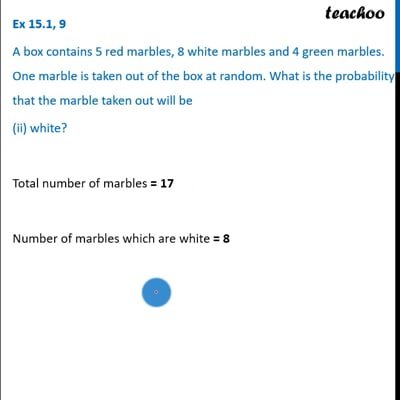This video is only available for Teachoo black users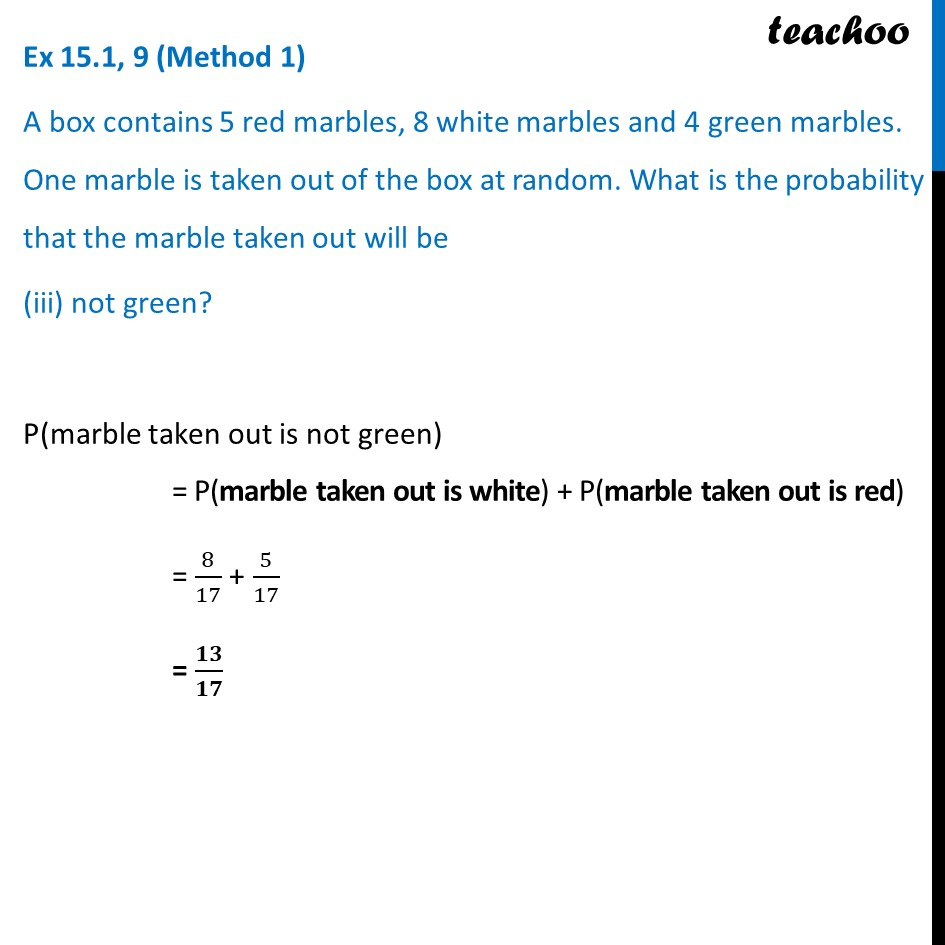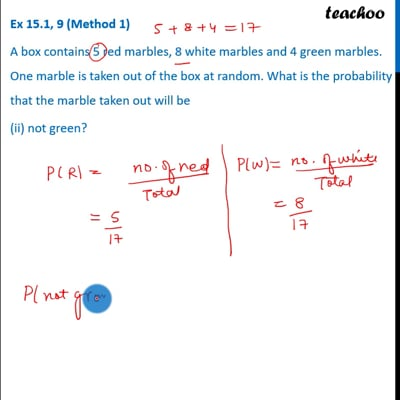This video is only available for Teachoo black users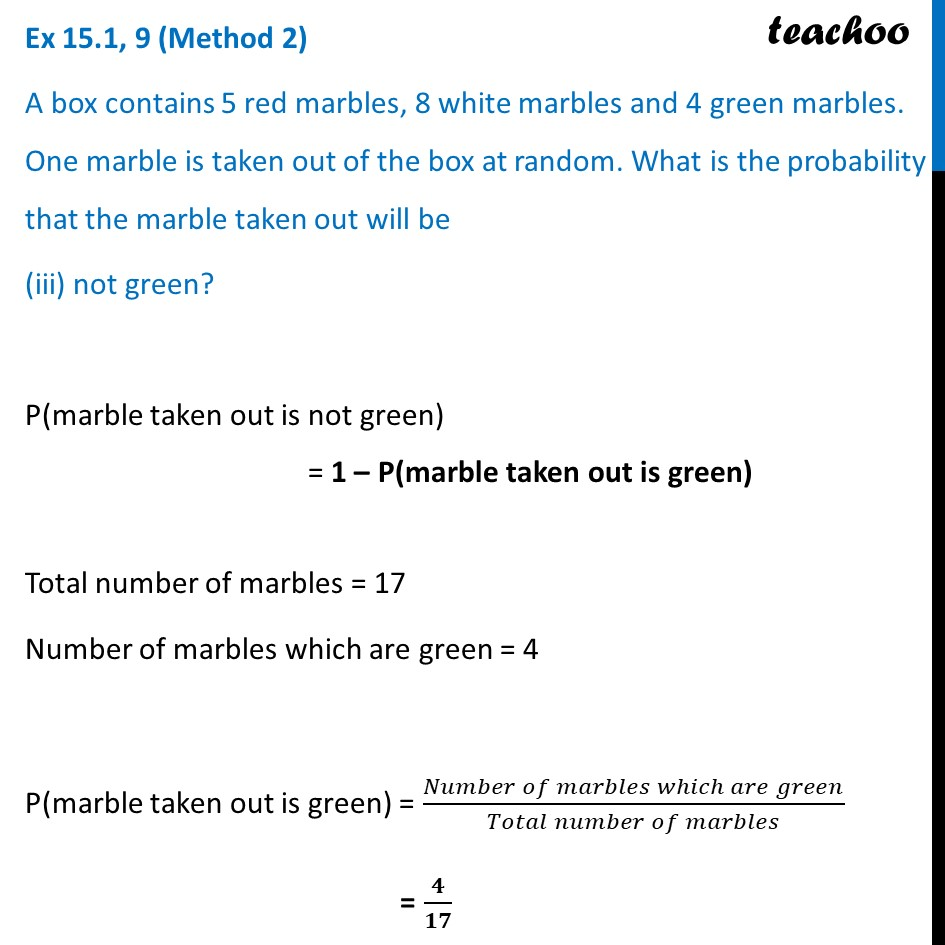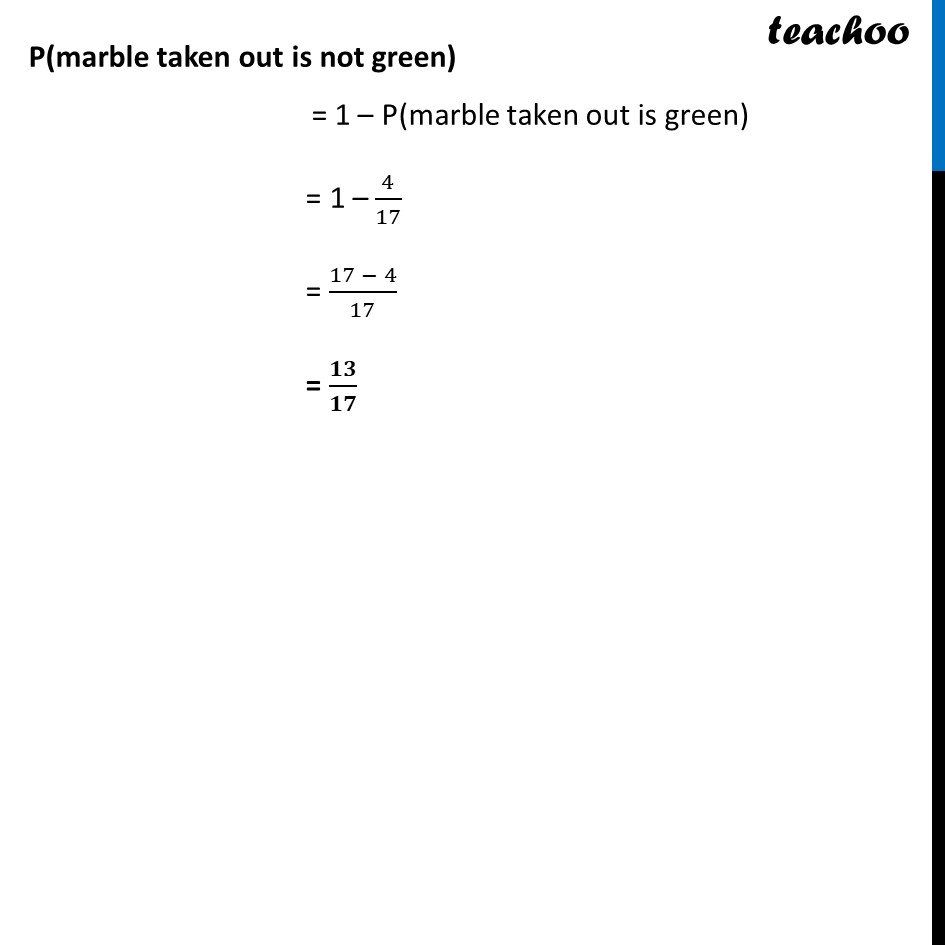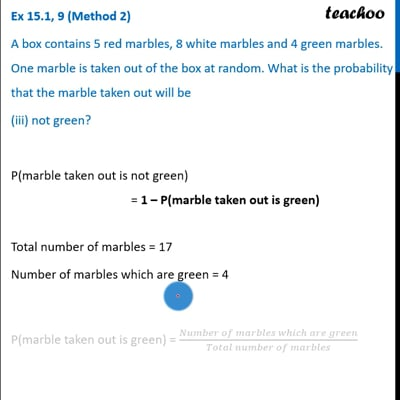This video is only available for Teachoo black users

Introducing your new favourite teacher - Teachoo Black, at only ₹83 per month

### Transcript

Ex 15.1, 9 A box contains 5 red marbles, 8 white marbles and 4 green marbles. One marble is taken out of the box at random. What is the probability that the marble taken out will be (i) red? Total number of marbles = 5 + 8 + 4 = 17 Number of marbles which are red = 5 P(marble taken out is red) = (𝑁𝑢𝑚𝑏𝑒𝑟 𝑜𝑓 𝑚𝑎𝑟𝑏𝑙𝑒𝑠 𝑤ℎ𝑖𝑐ℎ 𝑎𝑟𝑒 𝑟𝑒𝑑)/(𝑇𝑜𝑡𝑎𝑙 𝑛𝑢𝑚𝑏𝑒𝑟 𝑜𝑓 𝑚𝑎𝑟𝑏𝑙𝑒𝑠) = 𝟓/𝟏𝟕 Ex 15.1, 9 A box contains 5 red marbles, 8 white marbles and 4 green marbles. One marble is taken out of the box at random. What is the probability that the marble taken out will be (ii) white? Total number of marbles = 17 Number of marbles which are white = 8 P(marble taken out is white) = (𝑁𝑢𝑚𝑏𝑒𝑟 𝑜𝑓 𝑚𝑎𝑟𝑏𝑙𝑒𝑠 𝑤ℎ𝑖𝑐ℎ 𝑎𝑟𝑒 𝑤ℎ𝑖𝑡𝑒)/(𝑇𝑜𝑡𝑎𝑙 𝑛𝑢𝑚𝑏𝑒𝑟 𝑜𝑓 𝑚𝑎𝑟𝑏𝑙𝑒𝑠) = 𝟖/𝟏𝟕 Ex 15.1, 9 (Method 1) A box contains 5 red marbles, 8 white marbles and 4 green marbles. One marble is taken out of the box at random. What is the probability that the marble taken out will be (iii) not green? P(marble taken out is not green) = P(marble taken out is white) + P(marble taken out is red) = 8/17 + 5/17 = 𝟏𝟑/𝟏𝟕 Ex 15.1, 9 (Method 2) A box contains 5 red marbles, 8 white marbles and 4 green marbles. One marble is taken out of the box at random. What is the probability that the marble taken out will be (iii) not green? P(marble taken out is not green) = 1 – P(marble taken out is green) Total number of marbles = 17 Number of marbles which are green = 4 P(marble taken out is green) = (𝑁𝑢𝑚𝑏𝑒𝑟 𝑜𝑓 𝑚𝑎𝑟𝑏𝑙𝑒𝑠 𝑤ℎ𝑖𝑐ℎ 𝑎𝑟𝑒 𝑔𝑟𝑒𝑒𝑛)/(𝑇𝑜𝑡𝑎𝑙 𝑛𝑢𝑚𝑏𝑒𝑟 𝑜𝑓 𝑚𝑎𝑟𝑏𝑙𝑒𝑠) = 𝟒/𝟏𝟕 P(marble taken out is not green) = 1 – P(marble taken out is green) = 1 – 4/17 = (17 − 4)/17 = 𝟏𝟑/𝟏𝟕